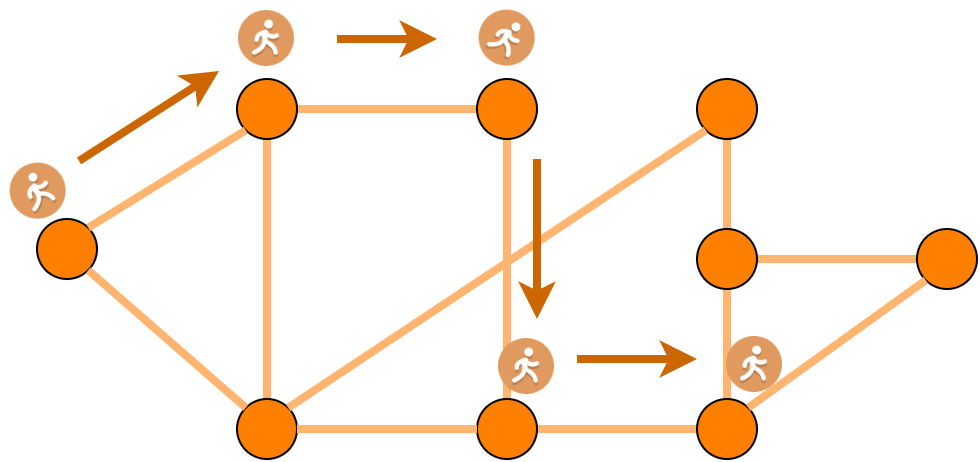# An Intuitive Explanation of DeepWalk

Source: Deep Learning on Medium# Introduction

This story is the second of the series Different Methods in Geometric Deep Learning. In the first one, we have made a gentle introduction to networks and geometric deep learning (GDL) by expressing its advantages over traditional ML approaches. Here is the link, in case you are interested.

In this story, we will discuss a pioneering method called DeepWalk, which uses language modeling approaches to learn node embeddings via leveraging the local structures in the network. With node embeddings at hand, we can use appropriate ML methods for GDL tasks such as node classification, link prediction, and community detection tasks.

DeepWalk is a two-stage method. In the first stage, it traverses the network with random walks to infer local structures by neighborhood relations. In the second stage, it uses an algorithm called SkipGram to learn embeddings that are enriched by the inferred structures. In the following sections, we first describe the two stages and then emphasize the advantages of DeepWalk.

# Stage 1 — Local Structure Discovery

In this stage, our goal is to discover neighborhoods in the network. To do so, we generate a fixed number (k) of random walks starting at each node. The length of each walk (l) is pre-determined. Thus, when this stage is finished, we obtain k node sequences of length l. The gist of random walk generation is the following assumption:

Adjacent nodes are similar and should have similar embeddings.

Based on this assumption, we will accept nodes that co-occur in a path as similar nodes. This means that the frequency of co-occurrence in random walks is an indicator of node similarity. Note that this is a reasonable assumption since edges, by design, mostly denote similar or interacting nodes in a network.

Here the effect of k and l is important. The more k is increased, the more the network is explored since more random walks are produced. On the other hand, when l is increased, paths become longer and more distant nodes are accepted as similar nodes. This corresponds to relaxing the similarity constraint and can introduce noisy and misleading co-occurrences.

# Stage 2 — SkipGram

SkipGram algorithm is a popular technique used to learn word embeddings. It was introduced by Mikolov et. al. in their well-known paper on word2vec. Given a corpus and a window size, SkipGram aims to maximize the similarity of word embeddings’ of the words that occur in the same window. These windows are also called context in NLP. This idea is based on the assumption that:

Words that occur in the same context, tend to have close meanings. Therefore, their embeddings should be close to each other as well.

To adapt SkipGram to networks, we need to determine what context corresponds to in the network world. This is where the random walks come into play. We can think of each walk produced in the previous step as a context or word window in a text. Thus, we can maximize the similarities of embeddings of nodes that occur on the same walks.

In this sense, node sequences in networks correspond to word sequences in text.

To learn the embeddings via SkipGram, we first generate random vectors of dimension d for each node. Secondly, we iterate over the set of random walks and update the node embeddings by gradient descent to maximize the probability of the neighbors of a node, given the node itself. This can be achieved by the softmax function. When all of the walks are processed, we can either continue optimization with additional passes over the same walk set or generate new walks on the network.

# Discussion

Assume a network with node features and a node classification task. With traditional ML techniques, we can learn feature to label mappings by assuming that each instance is independent of each other. Clearly, this is an invalid assumption for network structured data.

Thanks to DeepWalk, we can reflect neighborhood relations and local structures in the network to node representations. Since SkipGram tries to maximize similarities of neighboring nodes, nodes are sharing information between each other. This means that we enrich our existing feature set with node embeddings that were learned in a self-supervised manner.

Having learned new representations for nodes, we can now use classification models for our task. Yet, now we have got rid of an invalid assumption and introduced more information to our classifier. In the paper, authors have run extensive experiments to prove that DeepWalk embeddings facilitate classification performance for several tasks. They have also run parameter sensitivity experiments to observe the influence of each parameter on the embedding quality.

# Conclusion

In this story, we presented an intuitive explanation of DeepWalk, which is a method to learn node embeddings. DeepWalk borrows ideas from language modeling and incorporates them with network concepts. Its main proposition is that linked nodes tend to be similar and they should have similar embeddings as well. Despite more successful and efficient algorithms for node embedding exist nowadays, DeepWalk is prominent for the intuitive ideas it presented and its pioneering role.

 DeepWalk

 SkipGram